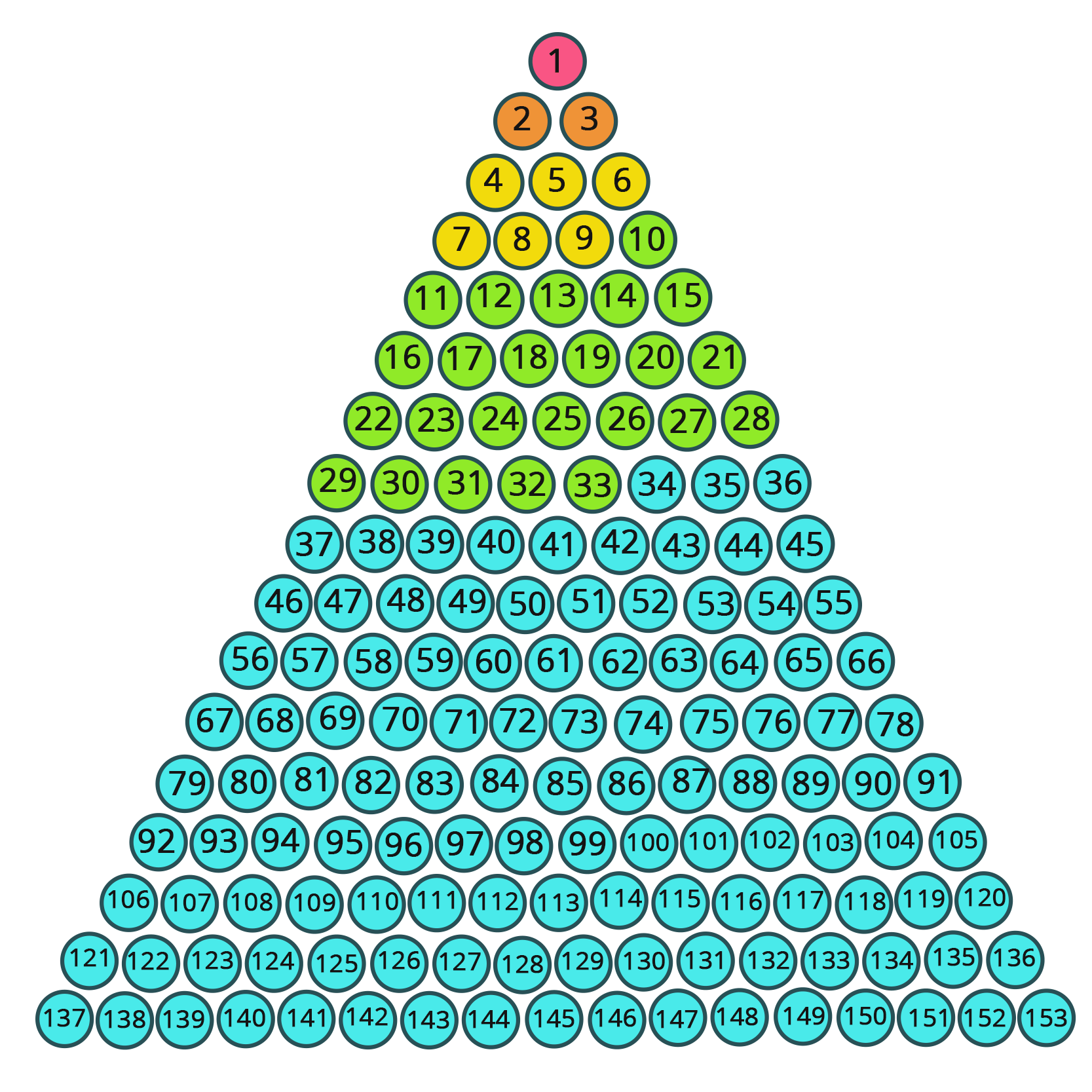• Call Now

1800-102-2727•

# Triangular Numbers

A triangular number is the sequence of numbers that are represented in the form of an equilateral triangle arranged in a series. These numbers can be demonstrated in a sequence of 1, 3, 6, 10, 15, 21, 28, etc. they can be marked in the form of dots which form a triangular pattern. The addition of previous numbers and order of succeeding numbers results in the array of triangular numbers. This article talks about triangular number sequences in a detailed manner.

A triangular number (demonstrated as Tn) can be illustrated as a dot pattern which is arranged in an equilateral triangle. They are defined as the series of numbers that can be attained as a result of summing the natural numbers. These triangular numbers are said to be figurative.

The following are the broad list of triangular numbers: 0, 1, 3, 6, 10, 15, 21, 28, 36, 45, 55, 66, 78, 91, 105, 120,136, 153, 171, 190, 210, 231, 253, 276, 300, 325, 351, 378, 406, 435, 465, 496, 528, 561, 595, 630, 666, 703, 741, 780, 820, 861, 903, 946, 990, 1035, 1081, 1128, 1176, 1225, 1275, 1326, 1378 etc.## Sum of a Triangular Number

In a triangular number pattern, the next number in the order can be added with an extra row. In detail,

• Consider the initial number 1 (one dot).
• While obtaining number 2, a new row is added and it contains two more dots to it.
• At number 3, three more dots are added which makes it look like an equilateral triangle.
• Likewise, for 4, another row that has four dots is added to the 3rd row.
• This process goes on until infinity.

So the sequence can be formed like this,

1, 1 + 2, 1 + 2 + 3, 1 + 2 + 3 + 4, 1 + 2 + 3 + 4 + 5, 1 + 2 + 3 + 4 + 5 + 6 etc.

Given below is the formula for triangular number sequence. This form can also be considered as 1st degree formula,In the above equation, n + 1/2 is called the binomial coefficient. It demonstrates the number of distinct pairs which can be selected from N + 1 objects.From the above formula, it can be understood that the summation of n natural numbers can help obtain a triangular number. In simple terms, one can say that the triangular numbers are the continued addition of natural numbers. If we take the sum of two consecutive natural numbers, we can effortlessly obtain a square number.From this formation, it can be observed that all even perfect numbers are triangular numbers.Talk to our expert
Resend OTP Timer =
By submitting up, I agree to receive all the Whatsapp communication on my registered number and Aakash terms and conditions and privacy policy